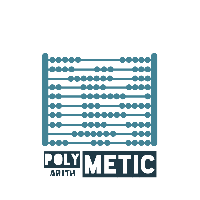Polymetic  1.1 A c++ library for polynomial and matrix arithmetic, focused on applications in Kinematics.
File List
Here is a list of all files with brief descriptions:
[detail level 12]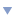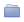include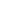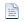gmpsupport.hpp The file contains explicit template declarations for gmp types as the template argument for polynomialsMatrix.hpp Contains typedefs and convinience operators which are wrappers around boost::numeric::ublas::matrix classMatrix.ipp Single file which is needed to be included to access all the functionallities associated with Matrix (which right now is boost::numeric::ublas::matrix)Matrix_impl.ipp Contains implementation of the template functionsMatrixAlgorithms.hpp Declarations for algorithms that work on MatricesMatrixAlgorithms.ipp Single header file which sould be included to access all the user defined algorithms which work on MatrixMatrixAlgorithms_impl.ipp Implements the template classes and functions in MatrixAlgorithms.hppPolynomial.hppPolynomial.ipp The single header file to include all the functionallities associated with the Polynomial classPolynomial_impl.ipp Implementation of Polynomial template class. Does not include implementation of the * operatorPolynomialMultiplication.ippPolynomialMultiplicationInterface.hppPolynomialMultiplicationInterface_impl.ippPolynomialMultiplicationSimple.hppPolynomialMultiplicationSimple.ipp Contains implementation of the template class PolynomialMultiplicationSimplesrcboost_matrix.cppdemo.cppgmp_integer_demo.cppgmp_integer_vector.cppPolynomial.cpp The file contains the explicit intantiations of the template class PolynomialtestPolynomial_testutils.ipp Header for the utility functions like == operator for polynomialsTest_Matrix_Operations.cpp Contains the tests for the matrix class with polynomial entries and arithmetic operations on thatTest_MatrixAlgorithms_double.cpp Contains the tests for the external/user defined functions like exp(), det(), etc,. on Matrix with double entriesTest_MatrixAlgorithms_polynomial_double.cppTest_Polynomial.cpp Contains the tests for the class Polynomial with field type as "double"Test_Polynomial_gmpz.cpp Contains the tests for the class Polynomial with field type as "mpz_class" which is the integer class from GMP